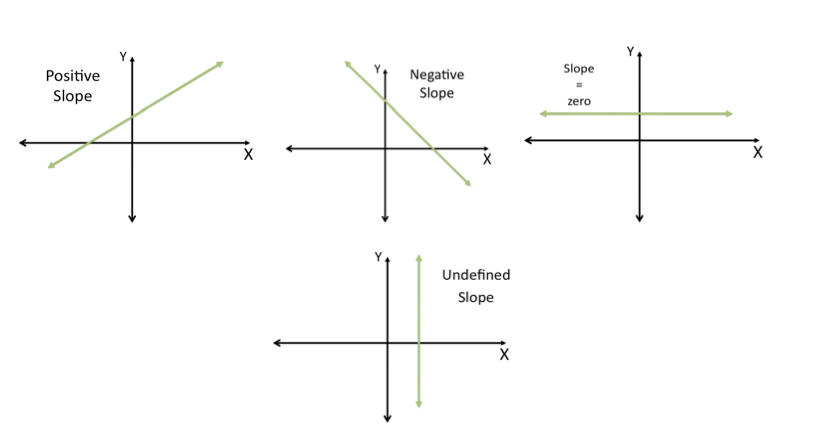How to find the Slope

If we have the equation y=mx + b, the value next to the X (m), is the slope of the line.
For example:

Y= 3X + 4, then the slope is 3 (m=3)
Y= -2x + 1, that is m=-2
Y= -0.5X + 1, that is m=-0.5 or m=-1/2
Y=1+ 9X, that is m=9

If the equation looks like this: 4X + Y = 1, you need to solve for Y.
For example,
Y= 1 -4X, therefore the slope is -4, that is m= -4

Types of Slopes

Below you can see the different types of slopes: